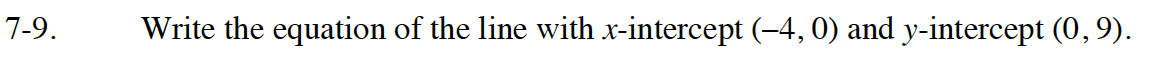### Home > CC4 > Chapter 7 > Lesson 7.1.1 > Problem7-9

7-9.

Write the equation of the line with x-intercept (–4, 0) and y-intercept (0, 9). Homework Help ✎Use the slope-intercept form of a line (y = mx + b).

Substitute the y-coordinate of the y-intercept for b in the equation.

Find the slope using any method you like, then substitute that value for m to finish writing the equation.